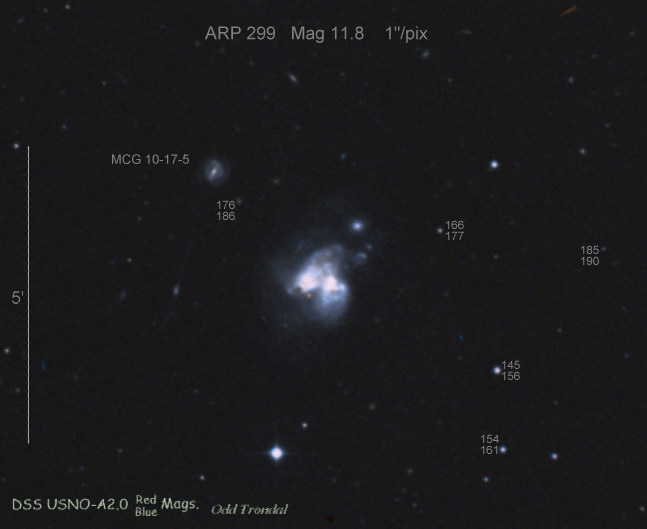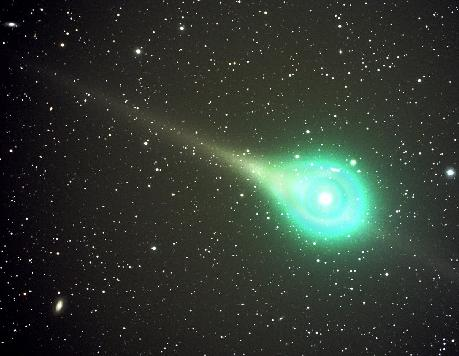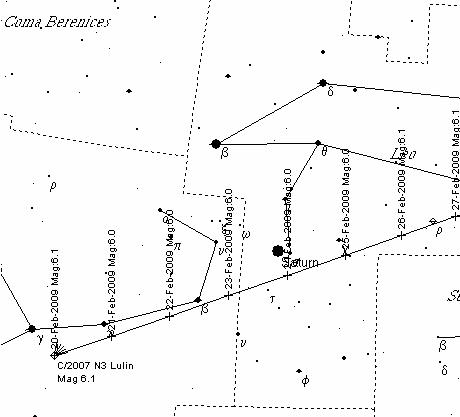## Ten photons per hourMarch 23, 2009

Posted by dorigo in astronomy, games, mathematics, personal, physics, science.
Tags: , , ,

Every working day I walk for about a mile to my physics department in Padova from the train station in the morning. I find it is a healthy habit, but I sometimes fear it also in some sense is a waste of time: if I catched a bus, I could be at work ten minutes earlier. I hate losing time, so I sometimes use the walking time to set physics problems to myself, trying to see whether I can solve them by heart. It is a way to exercise my mind while I exercise my body.

Today I was thinking at the night of stargazing I treated myself with last Saturday. I had gone to Casera Razzo, a secluded place in the Alps, and observed galaxies for four hours in a row with a 16″ dobsonian telescope, in the company of four friends (and three other dobs). One thing we had observed with amazement was a tiny speck of light coming from the halo of an interacting pair of galaxies in Ursa Major, the one pictured below.The small speck of light shown in the upper left of the picture above, labeled as MGC 10-17-5, is actually a faint galaxy in the field of view of NGC3690. It has a visual magnitude of +15.7: this is a measure of its integrated luminosity as seen from the Earth. It is a really faint object, and barely at the limit of visibility with the instrument I had. The question I arrived at formulating to myself this morning was the following: how many photons did we get to see per second through the eyepiece, from that faint galaxy ?

This is a nice, simple question, but computing its answer by heart took me the best part of my walk. My problem was that I did not have a clue of the relationship between visual magnitude and photon fluxes. So I turned to things I did know.Some background is needed to those of you who do not know how visual magnitudes are computed, so I will make a small digression here. The scale of visual magnitude is a semi-empirical one, which sets the brightest stars at magnitude zero or so, and defines a decrease of luminosity by a factor 100 per every five magnitudes difference. The faintest stars visible with the naked eye in a moonless night are of magnitude +6, and that means they are about 250 times fainter than the brightest ones. On the other hand, Venus shines at magnitude -4.5 at its brightest -almost 100 times as bright as the brightest stars-, and our Sun shines at a visual magnitude of about -27, more than a billion times brighter than Venus. The magnitude difference between two objects is in a relation with their relative brightness by a power law:$L_1/L_2 = 2.5^{-M_1+M_2}$; the factor 2.5 is an approximation for the fifth root of 100, and it corresponds to the brigthness ratio of two objects that differ by one unit of visual magnitude.

Ok, so we know how bright is the Sun. Now, if I could get how many photons reach our eye from it every second, I would make some progress. I reasoned that I knew the value of the solar constant: that is the energy radiated by the Sun on an area of 1 square meter on the ground of the Earth. I remembered a value of about 1 kilowatt (it is actually 1.366 kW, as I found out later in wikipedia).

Now, how many photons of visible light arriving per second on that square meter of ground correspond to 1 kilowatt of power ? I reasoned that I did not remember the energy of a single visible photon -I remembered it was in the electron-Volt range but I was not really sure- so I had to compute it.

The energy of a quantum of light is given by the formula$E = h \nu$, where$h$ is Planck’s constant and$\nu$ is the light frequency. However, all I knew was that visible light has a wavelength of about 500 nanometers (which is$5 \times 10^{-7} m$), so I had to use the more involved formula$E = hc/\lambda$, where now$c$ is the speed of light and$\lambda$ is the wavelength. I remembered that$h=6 \times 10^{-34} Js$, and that$c=3 \times 10^8 m/s$, so with some effort I could get$E=6 \times 10^{-34} \times 3 \times 10^8 / (5 \times 10^-7) = 4 \times 10^{-19}$, more or less.

My brains were a bit strained by the simple calculation above, but I was relieved to get back an energy roughly equal to that I expected -in the eV range (one eV equals$1.6 \times 10^{-19}$ Joules -that much I do know).

Now, if the Sun radiates 1 kW of power, which is a thousand Joules per second, how many visible photons do we get ? Here there is a subtlety I did not even bother considering in my walk to the physics department: only about half of the power from the Sun is in the form of visible light, so one should divide that power by two. But I was unhindered by this in my order-of-magnitude walk-estimate. Of course, 1 kW divided by$4 \times 10^{-19}$ makes$2.5 \times 10^{21}$ visible quanta of light per square meter per second.

Now, visual magnitude is expressed as the amount of light hitting the eye. A human eye has a surface of about 20 square millimeters, which is 20 millionths of a square meter: so the number of photons you get by looking straight at the sun (do not do it) is$1.2 \times 10^{14}$ per second. That’s a hundred trillions of ’em photons per second!

I was close to my goal now: the magnitude of the speck of galaxy I saw on Saturday is +15.7, the magnitude of the Sun is -27, so the difference is 43 magnitudes. This corresponds to$2.5^{43}$, which you might throw up your hands at, until you realize that every 5 units of the exponent the number increases by 100, so you just do$100^{43/5}$ which is$100^{8.6}$ which is$10^{17.2}$… Simple, isn’t it ?

Now, taking the number of photons reaching the eye from the Sun every second, and dividing by the ratio of apparent luminosities of the Sun and the galaxy, I could get$N_{\gamma}=10^{14} / 10^{17} = 10^{-3}$. One photon every thousand seconds!

Let me stress this: if you watch that patch of sky at night, the number of photons you get from that source alone is a few per hour! With my dobson telescope, which intensifies light by almost 10,000 times, I could get a rate of a few tens of photons per second, and the detail was indeed detectable!

If you are intested in the exact number, which I worked out after reaching my office and the tables of constants in the PDG booklet, I computed a rate of$N_{\gamma}=3.4 \times 10^{-3}$ photons per second with unaided eye, and 22 per second through the eyepiece of the telescope. Without telescope, that galaxy sends to each of us about 10 photons per hour!

UPDATE: this post will remain as one clear example of how dangerous it is to compute by heart! Indeed, somewhere in my order-of-magnitude conversions above I dropped a factor 10^2 -which, mind you, is not horrible in numbers which have 20 digits or so; but when one wants to get back to reasonable estimates for reasonably small numbers, it does count a lot. So, after taking care of some other (more legitimate) approximations, if one computes things correctly, the number of photons from the galaxy seen with the unaided eye is more like two hundred per hour, and in the telescope it is of about 350 per second.

## Comet Lulin is a naked-eye object!February 19, 2009

Posted by dorigo in astronomy, news, science.
Tags: , , ,

Comet Lulin (C/2007 N3) is approaching the minimum distance from our planet – the conjunction will occur on February 24th at a distance of 61 million kilometers- and is already a naked-eye object in the sky, glowing at a visual magnitude of +5.6 with what is described as a bright green colour. The coma has a diameter of 20 arcminutes (two-thirds of the Moon’s diameter). As you can see from Jack Newton’s picture below, the comet shows both a tail and an anti-tail, with a bright oval coma.The conjunction is very convenient given the absence of any moonlight, and its position in the sky, almost perfectly in the opposite direction with respect to the Sun.  A pair of binoculars, even low-power ones, will reveal the comet easily from your back yard even in light polluted areas, while under dark skies you should be able to detect the comet even with the unaided eye; a telescope should be used with low magnification to show the comet in all its glory.  The object moves quickly in the sky, and its apparent motion is easy to detect if you have patience to observe the comet for a while.

You can find the comet in Libra today and tomorrow (check the map below -click to enlarge), while at conjunction on Feb 24th it will be in Leo, just a few degrees due South of Saturn. In a few days its brightness could increase by another magnitude (magnitudes in the chart are not necessarily correct).For a beautiful gallery of images of this beautiful comet, I advise you to visit the Spaceweather site.

## A naked-eye comet to brighten our nights next month!January 9, 2009

Posted by dorigo in astronomy, news, science.
Tags: ,